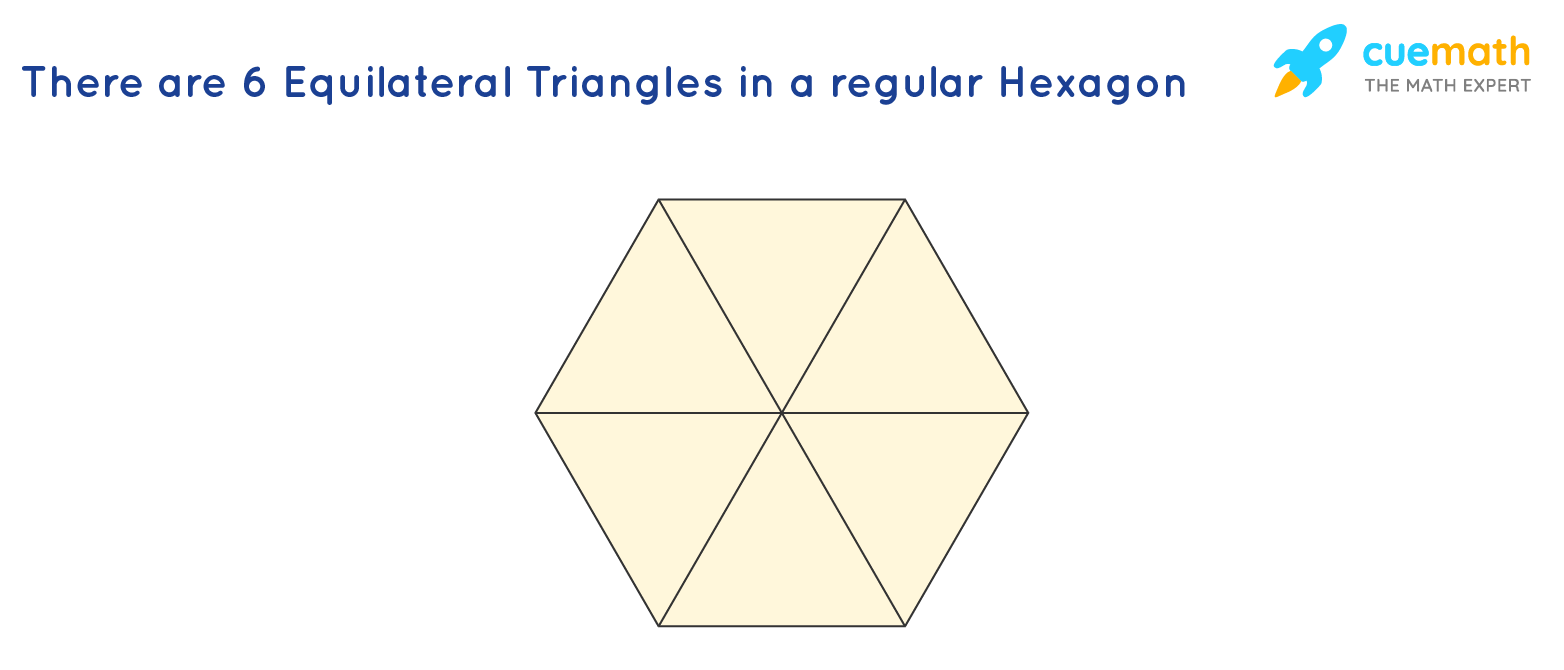# How Many Equilateral Triangles are there in a Regular Hexagon?

## Question: How many equilateral triangles are there in a regular hexagon?

A regular hexagon is a polygon with 6 equal sides and equal angles

## Answer: There are 6 equilateral triangles in a regular hexagon

Let's look into the structure of a regular hexagon with all the end to end vertices connected

## Explanation:

An equilateral triangle is a regular polygon with 3 equal sides with each angle equal to 60 degreesWe know that the sum of interior angles of a hexagon is 720°

Thus, each internal angle of a hexagon measures 120°                (since, 720/6 = 120)

When the lines are connected using two opposite vertices, the internal angles are bisected and hence, each angle is 60° in measure

The angle at the centre of the hexagon is 360° which is being divided into 6 equal parts

Thus, the value of each angle at the centre is equal to 60°

Hence, we see that all three angles of the 6 triangles formed in the given hexagon is equal to 60°

This proves that there are 6 equilateral triangles

We can thus form 6 equilateral triangles by connecting the opposite vertices of a regular hexagon